## Lattice PointA Point at the intersection of two or more grid lines in a ruled array. (The array of grid lines could be oriented to form unit cells in the shape of a square, rectangle, hexagon, etc.) However, unless otherwise specified, lattice points are generally taken to refer to points in a square array, i.e., points with coordinates, where,, ... are Integers.

An-D-lattice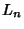lattice can be formally defined as a free-Module in complex-D space.

The Fraction of lattice points Visible from the Origin, as derived in Castellanos (1988, pp. 155-156), is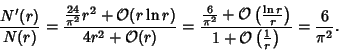Therefore, this is also the probability that two randomly picked integers will be Relatively Prime to one another.

For, it is possible to select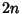lattice points with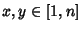such that no three are in a straight Line. The number of distinct solutions (not counting reflections and rotations) for, 2, ..., are 1, 1, 4, 5, 11, 22, 57, 51, 156 ... (Sloane's A000769). For large, it is conjectured that it is only possible to select at most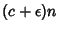lattice points with no three Collinear, where(Guy and Kelly 1968; Guy 1994, p. 242). The number of the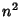lattice pointswhich can be picked with no four Concyclic is(Guy 1994, p. 241).

A special set of Polygons defined on the regular lattice are the Golygons. A Necessary and Sufficient condition that a linear transformation transforms a lattice to itself is that it be Unimodular. M. Ajtai has shown that there is no efficient Algorithm for finding any fraction of a set of spanning vectors in a lattice having the shortest lengths unless there is an efficient algorithm for all of them (of which none is known). This result has potential applications to cryptography and authentication (Cipra 1996).

See also Barnes-Wall Lattice, Blichfeldt's Theorem, Browkin's Theorem, Circle Lattice Points, Coxeter-Todd Lattice, Ehrhart Polynomial, Gauss's Circle Problem, Golygon, Integration Lattice, Jarnick's Inequality, Lattice Path, Lattice Sum, Leech Lattice, Minkowski Convex Body Theorem, Modular Lattice, N-Cluster, Nosarzewska's Inequality, Pick's Theorem, Poset, Random Walk, Schinzel's Theorem, Schröder Number, Visible Point, Voronoi Polygon

References

Apostol, T. Introduction to Analytic Number Theory. New York: Springer-Verlag, 1995.

Castellanos, D. The Ubiquitous Pi.'' Math. Mag. 61, 67-98, 1988.

Cipra, B. Lattices May Put Security Codes on a Firmer Footing.'' Science 273, 1047-1048, 1996.

Eppstein, D. Lattice Theory and Geometry of Numbers.'' http://www.ics.uci.edu/~eppstein/junkyard/lattice.html.

Guy, R. K. Gauß's Lattice Point Problem,'' Lattice Points with Distinct Distances,'' Lattice Points, No Four on a Circle,'' and The No-Three-in-a-Line Problem.'' §F1, F2, F3, and F4 in Unsolved Problems in Number Theory, 2nd ed. New York: Springer-Verlag, pp. 240-244, 1994.

Guy, R. K. and Kelly, P. A. The No-Three-in-Line-Problem.'' Canad. Math. Bull. 11, 527-531, 1968.

Hammer, J. Unsolved Problems Concerning Lattice Points. London: Pitman, 1977.

Sloane, N. J. A. Sequence A000769/M3252 in An On-Line Version of the Encyclopedia of Integer Sequences.'' http://www.research.att.com/~njas/sequences/eisonline.html and Sloane, N. J. A. and Plouffe, S. The Encyclopedia of Integer Sequences. San Diego: Academic Press, 1995.